# Plotting tools

## plotting RRphylo outputs onto the tree

Phenotypic and evolutionary rate values can be plotted onto the tree branches by means of `plotRR`. The function takes as mandatory objects the result of `RRphylo`, the vector/matrix of phenotypic values (`y`) used to generate the `RR` object, and a `multivariate` argument indicating whether individual rates for each variable (`"multiple.rates"`) or the norm2 vector of multivariate rates (`"rates"`) should be plotted. The output includes two customized functions to chart phenotipic values/evolutionary rates along the tree branches: `plotRRphen` and `plotRRrates`. The plots generated by both these functions are completely customizable by providing a list of `tree.args`, being all the arguments usually passed to the function `plot.phylo`, `color.pal` function to generate the color palette and a list of `colorbar.args` to be passed to the function `colorbar`. The only mandatory argument for `plotRRphen` and `plotRRrates` with multivariate data is the name or the number of the `variable` to plot.

``````### load the RRphylo example dataset including Apes tree and shape data (multivariate)
data("DataApes")
DataApes\$PCstage->PCstage
DataApes\$Tstage->Tstage

# Perform RRphylo
RRphylo(tree=Tstage,y=PCstage)->RR

# Visualize phenotypic values and "multiple" evolutionary rates onto the
# phylogenetic tree.
plotRR(RR,y=PCstage,multivariate="multiple.rates")->pRRmulti

# Visualize phenotypic values and the norm2 vector of multiple rates onto the
# phylogenetic tree.
plotRR(RR,y=PCstage,multivariate="rates")->pRR``````
``````# Using default options
pRRmulti\$plotRRphen(variable=1)
pRRmulti\$plotRRrates(variable=2)``````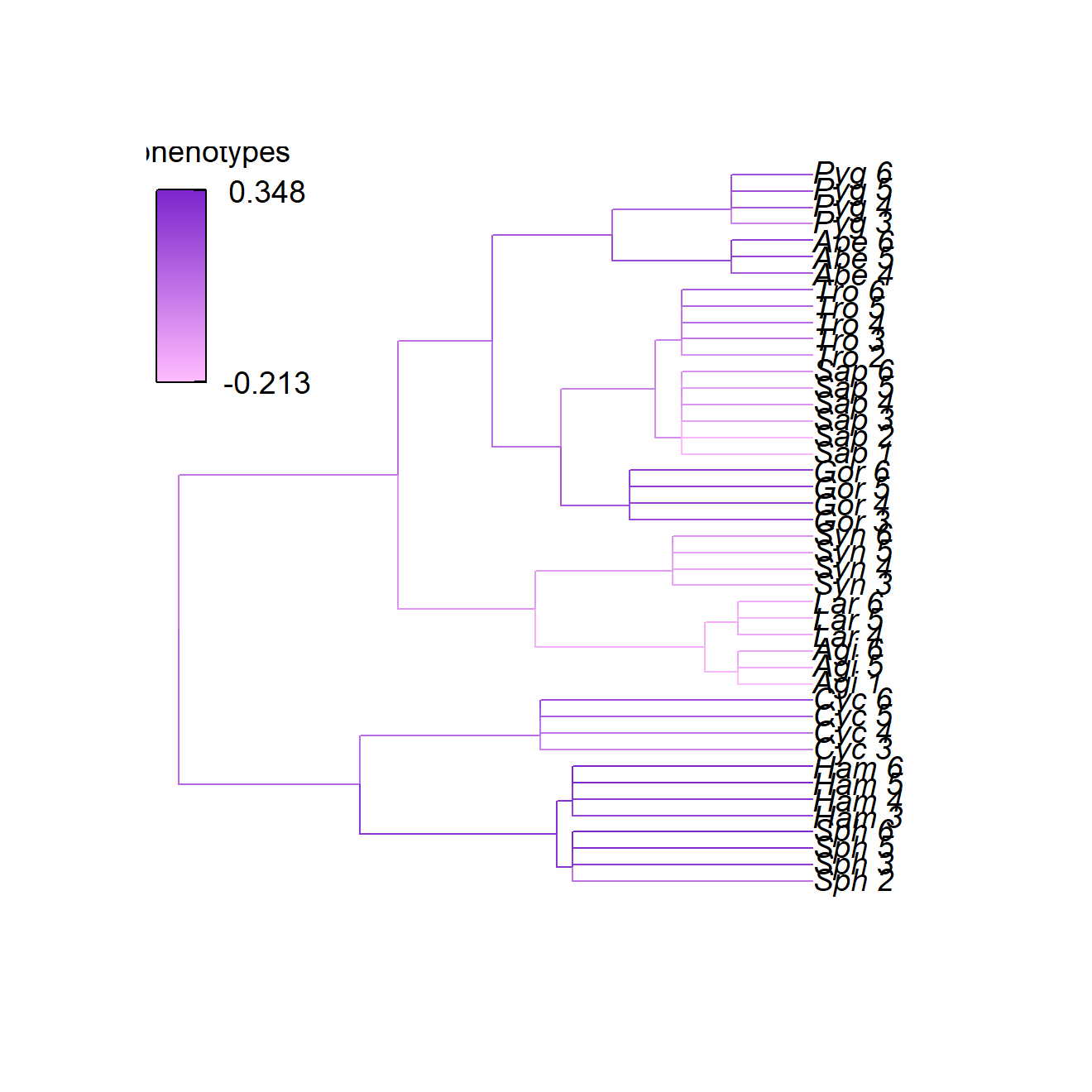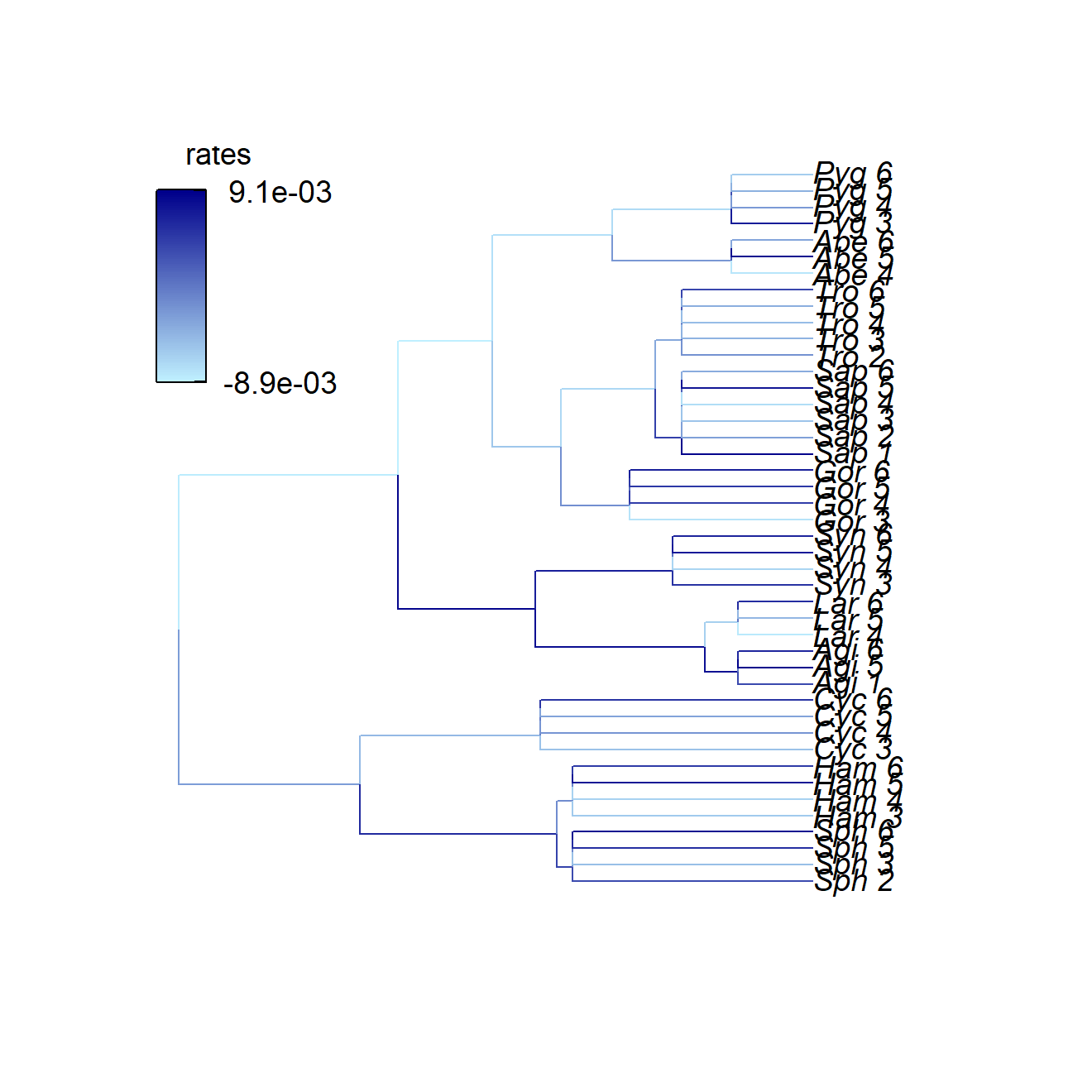``````# Using default options
pRR\$plotRRphen(variable=1) # this is the same as before of course
pRR\$plotRRrates()``````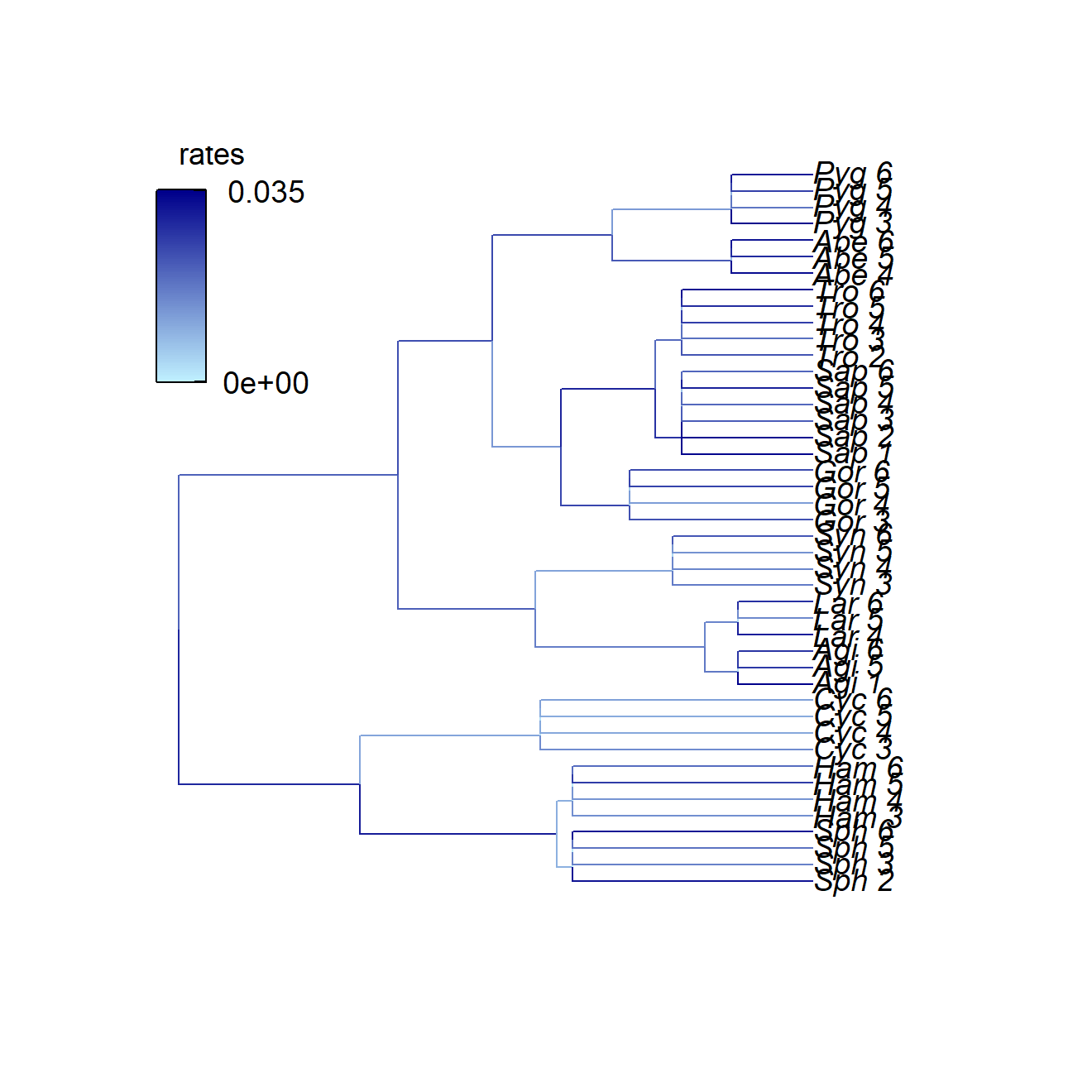``````# Customizing parameters
pRRmulti\$plotRRphen(variable=3,tree.args=list(edge.width=2,cex=0.7),color.pal=heat.colors,
pRRmulti\$plotRRrates(variable=4,tree.args=list(edge.width=2,direction="leftwards",cex=0.7),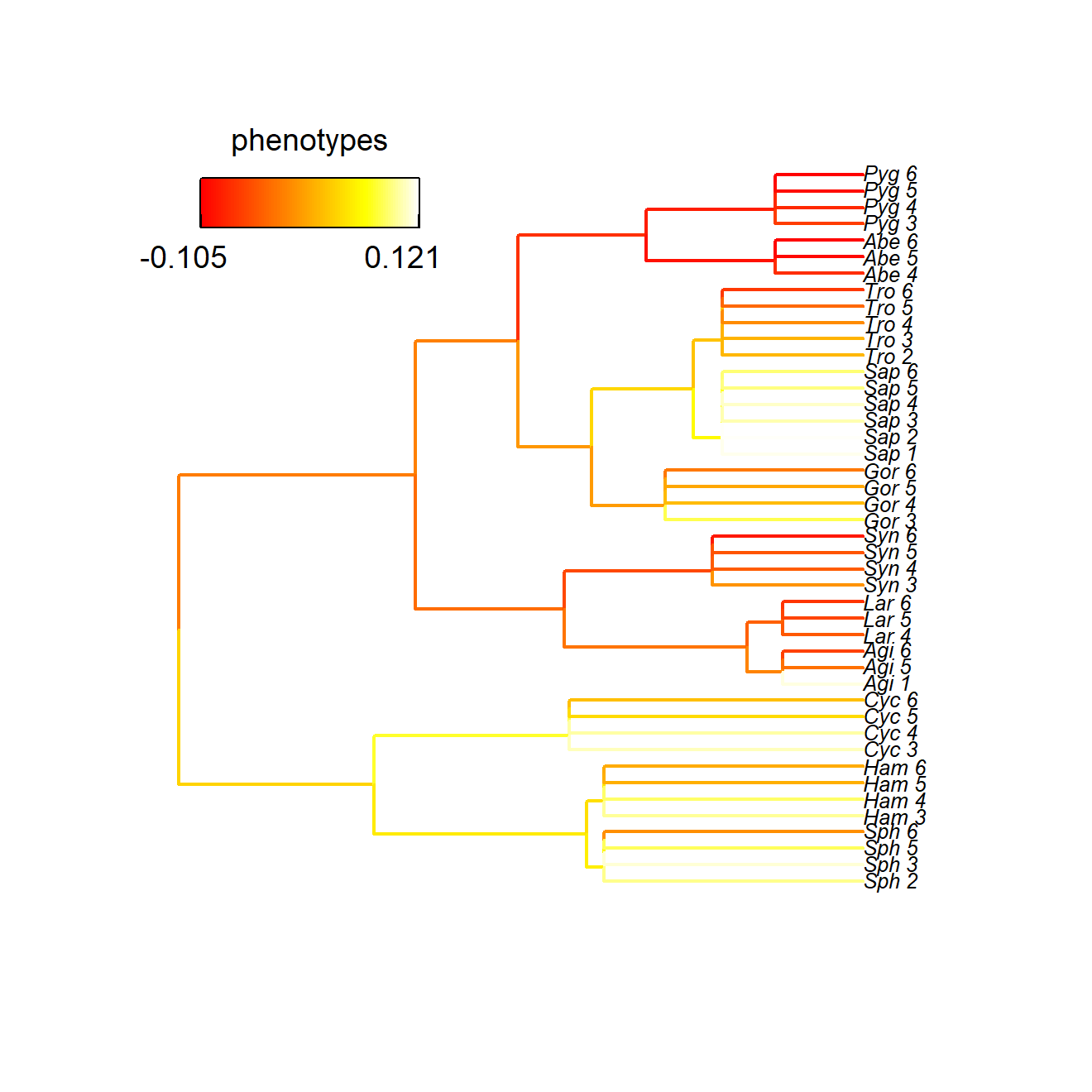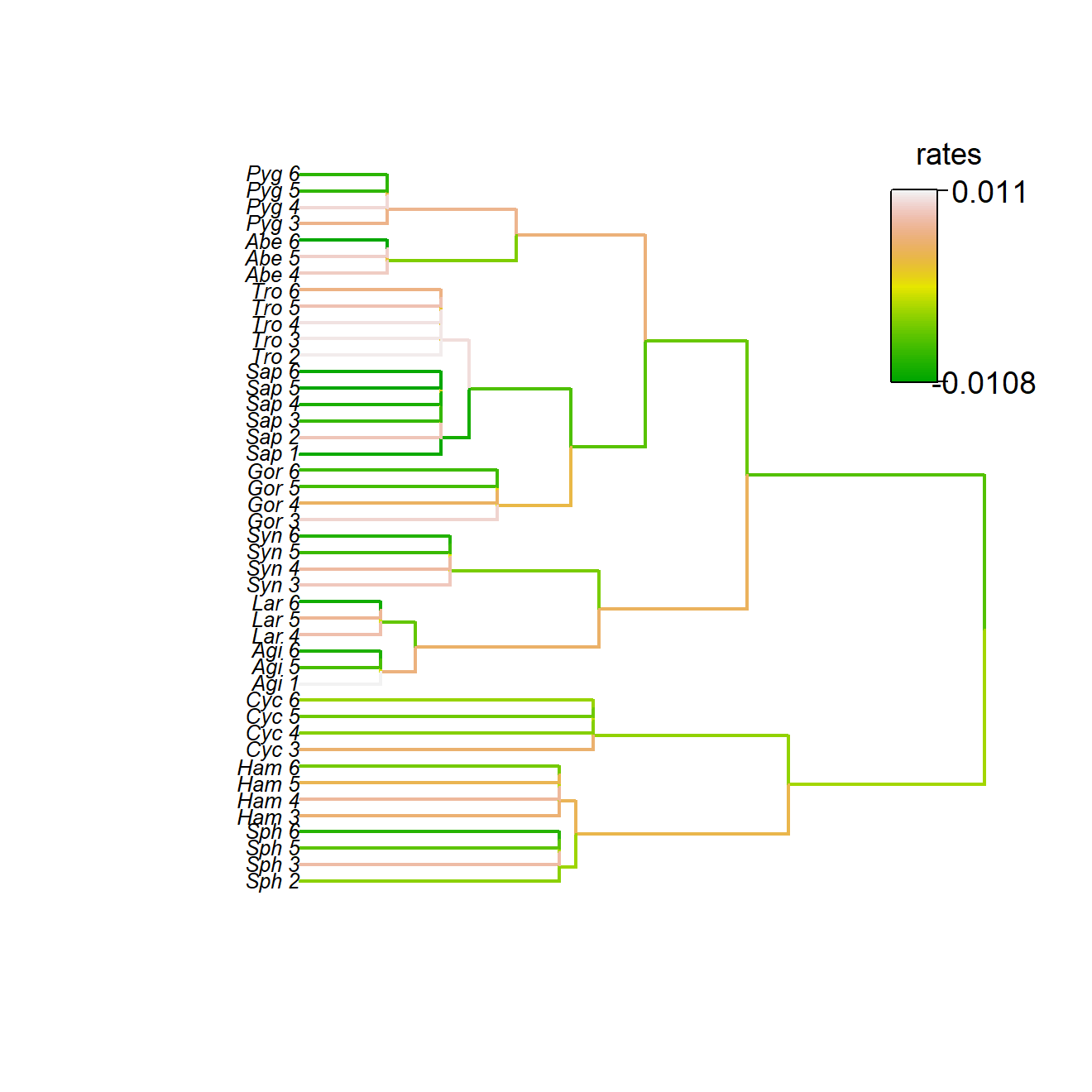## plotting RRphylo rates

Evolutionary rates computed by RRphylo for all the tree branches belonging to a specific clade can be visualized by means of `plotRates`. The function takes as objects the result of `RRphylo` and the focal `node` number (located on the tree returned by `RRphylo`) and returns two customized functions. The function `plotHist` plots the histograms of rates (in ln absolute values) computed for the focal clade compared to rates computed for the rest of the tree. The plot is completely customizable by providing lists of `hist.args`, being all the arguments usually passed to the function `hist`, and `legend.args`, usually passed to the function `legend`. The function `plotLollipop` returns a lollipop plot (see documentation for `lollipoPlot` function in RRphylo package) of rate values for each branch in the clade. As well as `plotHist`, this is customizable by indicating lists of `lollipop.args` for the lollipops and `line.args` for the vertical line representing the mean rate for the entire tree. Additionally, `plotLollipop` returns the vector of evolutionary rates for each branch within the focal clade, sorted in the same order they are plotted, that is from the largest to the smallest value. In this way, the user could ideally set different graphical parameters for each species/node in the vector (see the example below).

``````### load the RRphylo example dataset including Cetaceans tree and ln body masses
data("DataCetaceans")
DataCetaceans\$treecet->treecet
DataCetaceans\$masscet->masscet

# Perform RRphylo
RRphylo(treecet,masscet)->RRcet

# Visualize evolutionary rates computed for the clade including extant Mysticetes
plotRates(RRcet,node=131)->pRates``````
``````# Using default options
par(mar=c(3,3,1,1),mgp=c(1.7,0.5,0))
pRates\$plotHist()
par(mar=c(3,12,1,1),mgp=c(1.7,0.5,0))
pRates\$plotLollipop()->pLol``````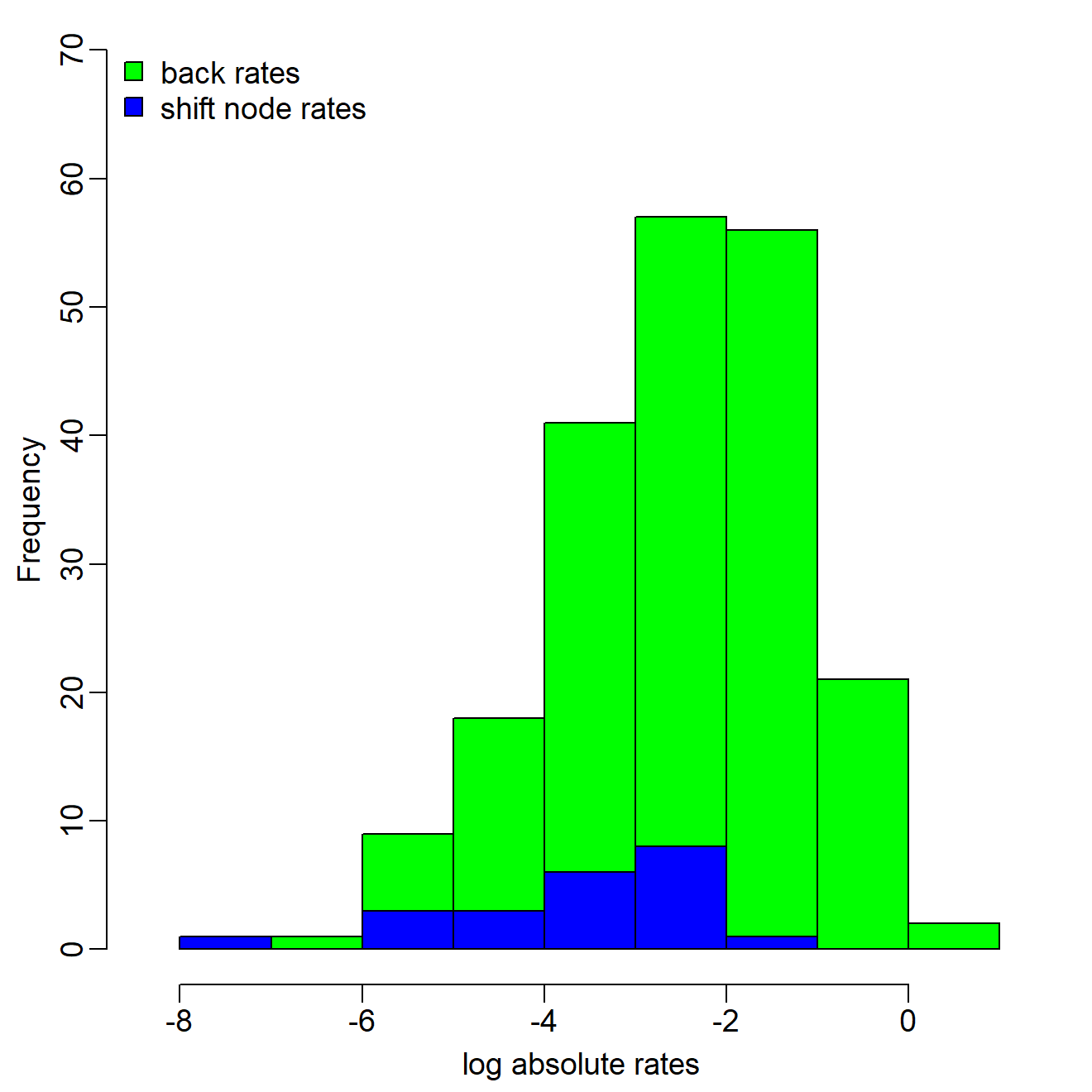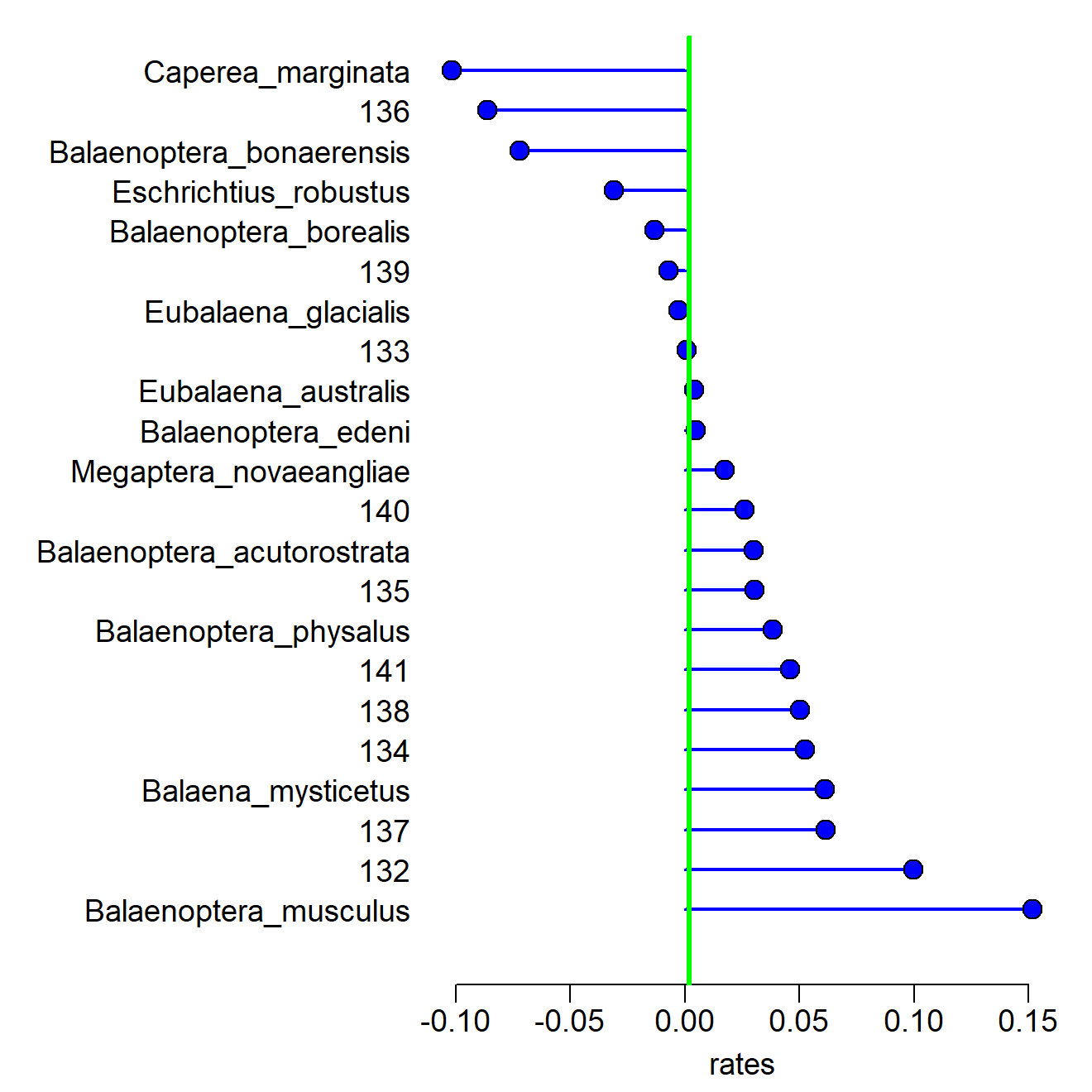``````# Customizing parameters
par(mar=c(3,3,1,1),mgp=c(1.7,0.5,0))
pRates\$plotHist(hist.args=list(yaxt="n",ylab=NULL,col1="blue",col2="cyan"),
legend.args = list(pch=21,pt.cex=2))

# Setting different pch and color for nodes and species
colo<-rep("gray70",length(pLol))
colo[names(pLol)%in%treecet\$tip.label]<-"cyan"
pchc<-rep(22,length(pLol))
pchc[names(pLol)%in%treecet\$tip.label]<-21

par(mar=c(3,12,1,1),mgp=c(1.7,0.5,0))
pRates\$plotLollipop(lollipop.args = list(pch=pchc,bg=colo,col=colo,cex=2,lwd=2),
line.args = list(col="deeppink2",lwd=4,lty=2))->pLol2``````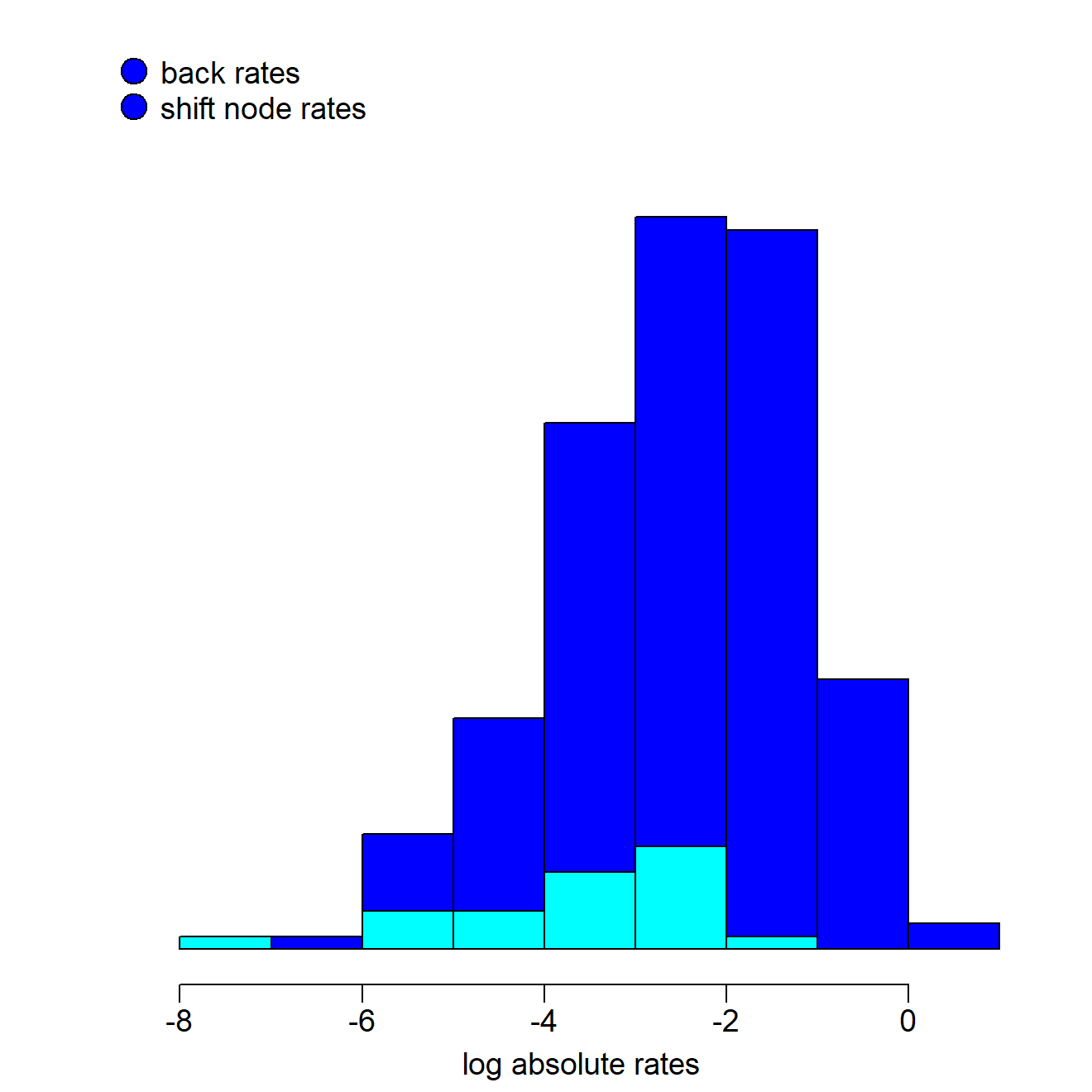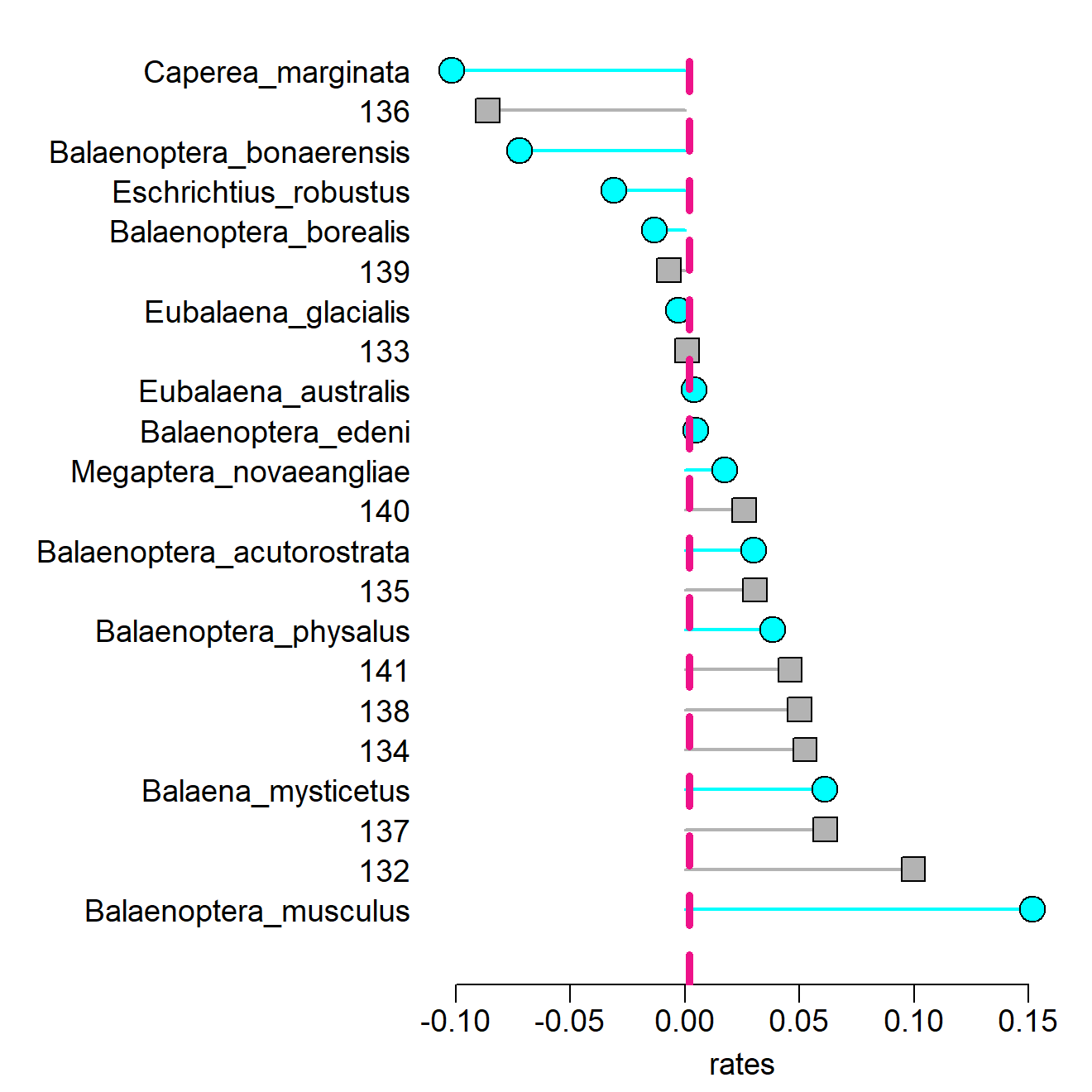## plotting search.trend results

Results for phenotypic and evolutionary rates trends through time returned by the function search.trend can be visualized by means of `plotTrend`. Such function takes as only object the output of `search.trend` and returns customized functions to produce plots of phenotypes (`plotSTphen`) and (rescaled) evolutionary rates (`plotSTrates`) versus time regressions on the entire tree. The plots generated by both these functions are basically made by a scatterplot representing the distribution of phenotypes/rates versus time, a polygon depicting the 95% CI of slopes generated according to the Brownian Motion (see search.trend for further details) and the regression line. Each of these elements can be customized by the user by specifying the arguments `plot.args`, `polygon.args`, and `line.args`. The only mandatory argument for `plotSTphen` and `plotSTrates` is the name or the number of the `variable` to plot.

``````### load the RRphylo example dataset including Felids tree and 4 mandible shape variables (PCs)
data("DataFelids")
DataFelids\$treefel->treefel
DataFelids\$PCscoresfel->PCscoresfel

# Perform RRphylo and search.trend
RRphylo(treefel,PCscoresfel)->RRfel
search.trend(RRfel,PCscoresfel)->ST

# Visualize search.trend results
plotTrend(ST)->pTrend``````
``````# Using default options
par(mfrow=c(1,2),mar=c(4,3,3,1),mgp=c(1.5,0.5,0))
pTrend\$plotSTphen("PC1")
pTrend\$plotSTrates(1) # This is for PC1 as well``````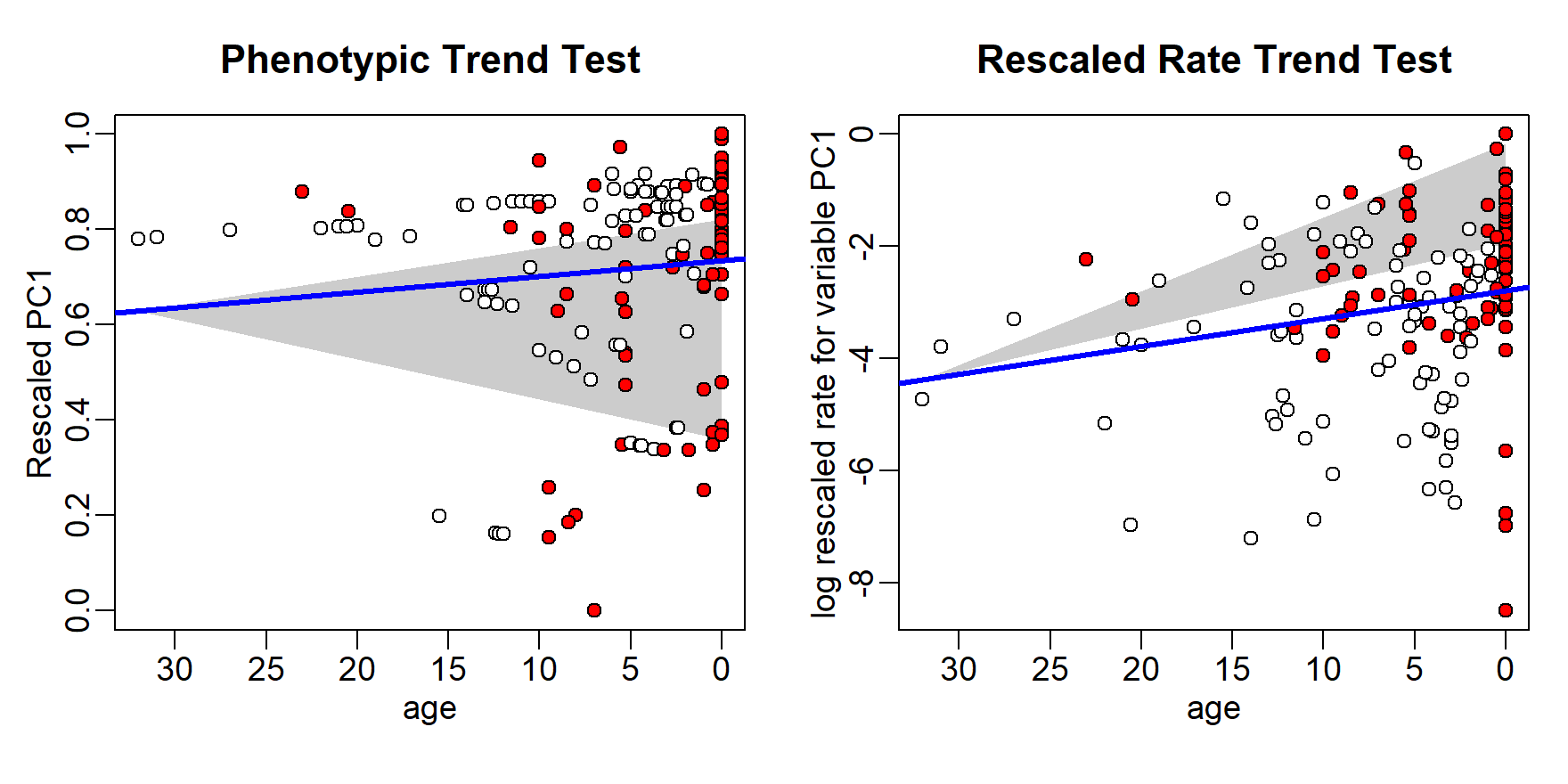``````
## Customizing parameters
# Extracting nodes and tips descending from the most recent common ancestor of Felinae
library(phytools)
Felinae<-getDescendants(treefel,94)
Felinae[which(Felinae<=Ntip(treefel))]<-treefel\$tip.label[Felinae[which(Felinae<=Ntip(treefel))]]

# Setting different pch for Felinae only
pchc<-rep(21,nrow(ST\$trend.data\$phenotypeVStime))
pchc[rownames(ST\$trend.data\$phenotypeVStime)%in%Felinae]<-6

par(mfrow=c(1,2),mar=c(4,3,3,1),mgp=c(1.5,0.5,0))
pTrend\$plotSTphen("PC2",plot.args = list(pch=pchc,cex=1.3),
polygon.args = list(col="white",border="black",lwd=2,lty=3),
line.args = list(lty=4,col="purple3"))

# When multivariate data are used, a "rate" vector is calculated as the 2-norm
# vector of rates computed for each individual variable. The "total rate" can be plotted:
pTrend\$plotSTrates("rate") # Equivalent to setting variable = 5``````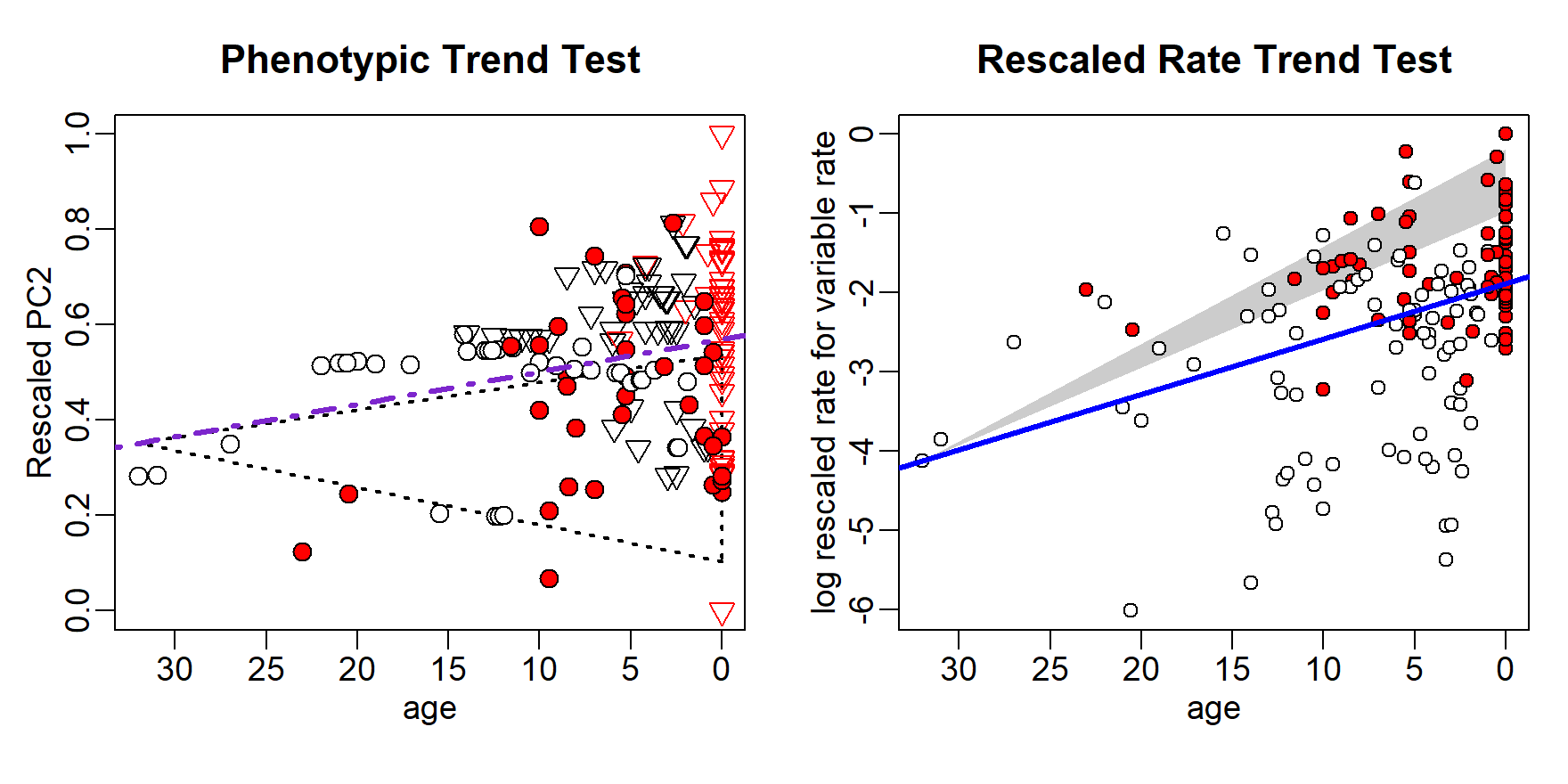If the output of `search.trend` also includes results for phenotypic and evolutionary rates trends trough time occurring at individual clades in the tree, `plotTrend` returns two additional functions to generate plots for individual nodes (`plotSTphenNode` and `plotSTratesNode`). As above, these functions allow the user to customize the plot by setting the parameters `plot.args` (for everything pertaining the scatterplot), `lineTree.args` and `lineNode.args` (for the regression lines for the entire tree and the clade, respectively), and `node.palette` (including as many colors as the number of nodes the plot should be produced for). Mandatory arguments are the `variable` and the indices or numbers of nodes to plot (up to 9 nodes at the same time). An argument peculiar to these functions (listed in `plot.args`) is `pch.node`, which can be used to set different pch for each node.

``````# Perform search.trend setting Smilodontini and Pantherini as individual clades

# Visualize search.trend results
``````# Using default options
par(mar=c(4,3,3,1),mgp=c(1.5,0.5,0))
pTrend2\$plotSTphenNode("PC1",node=1:2)``````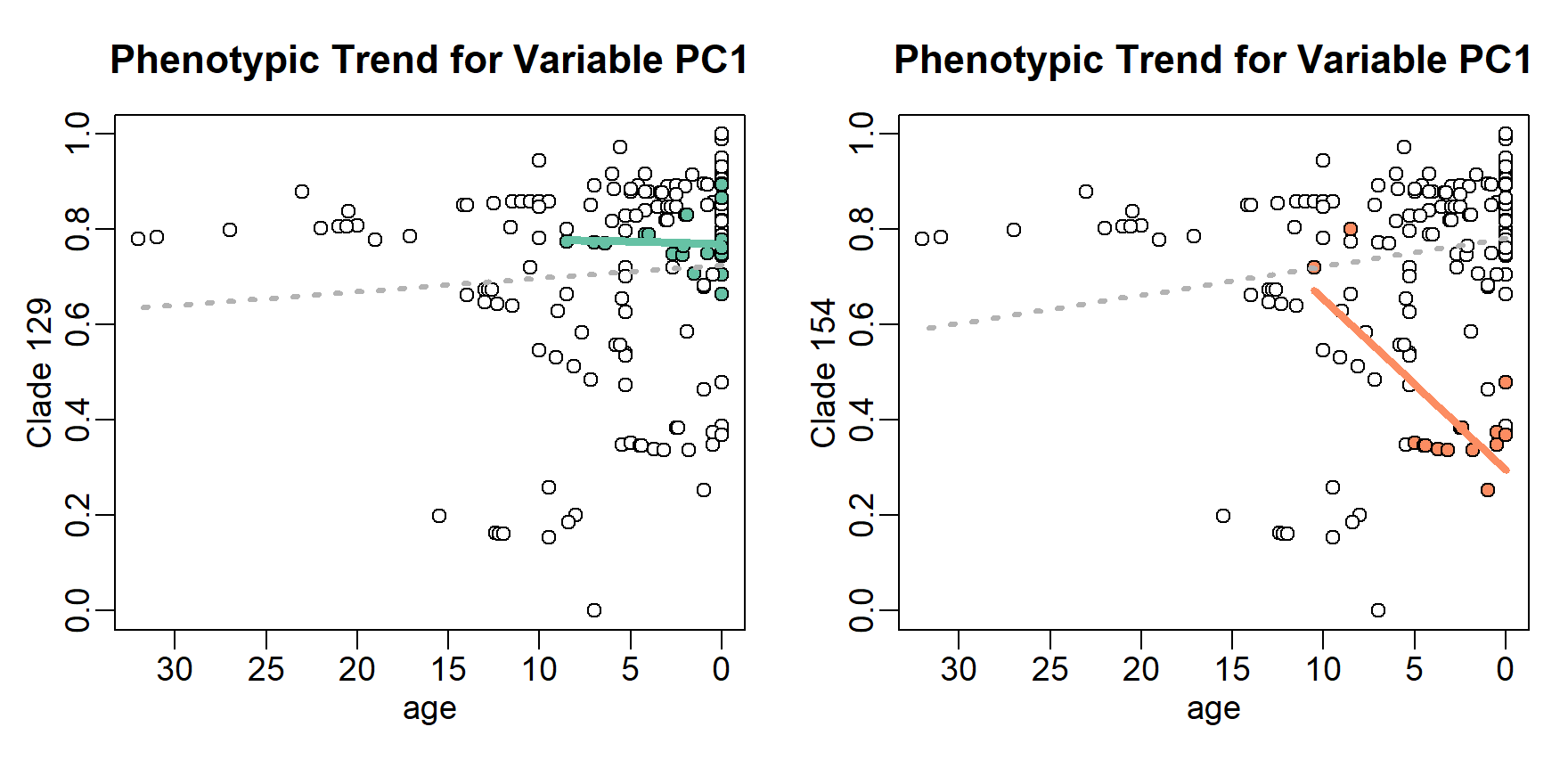``pTrend2\$plotSTratesNode("PC1",node=c(154,129)) # This is the same as indicating node= 2:1``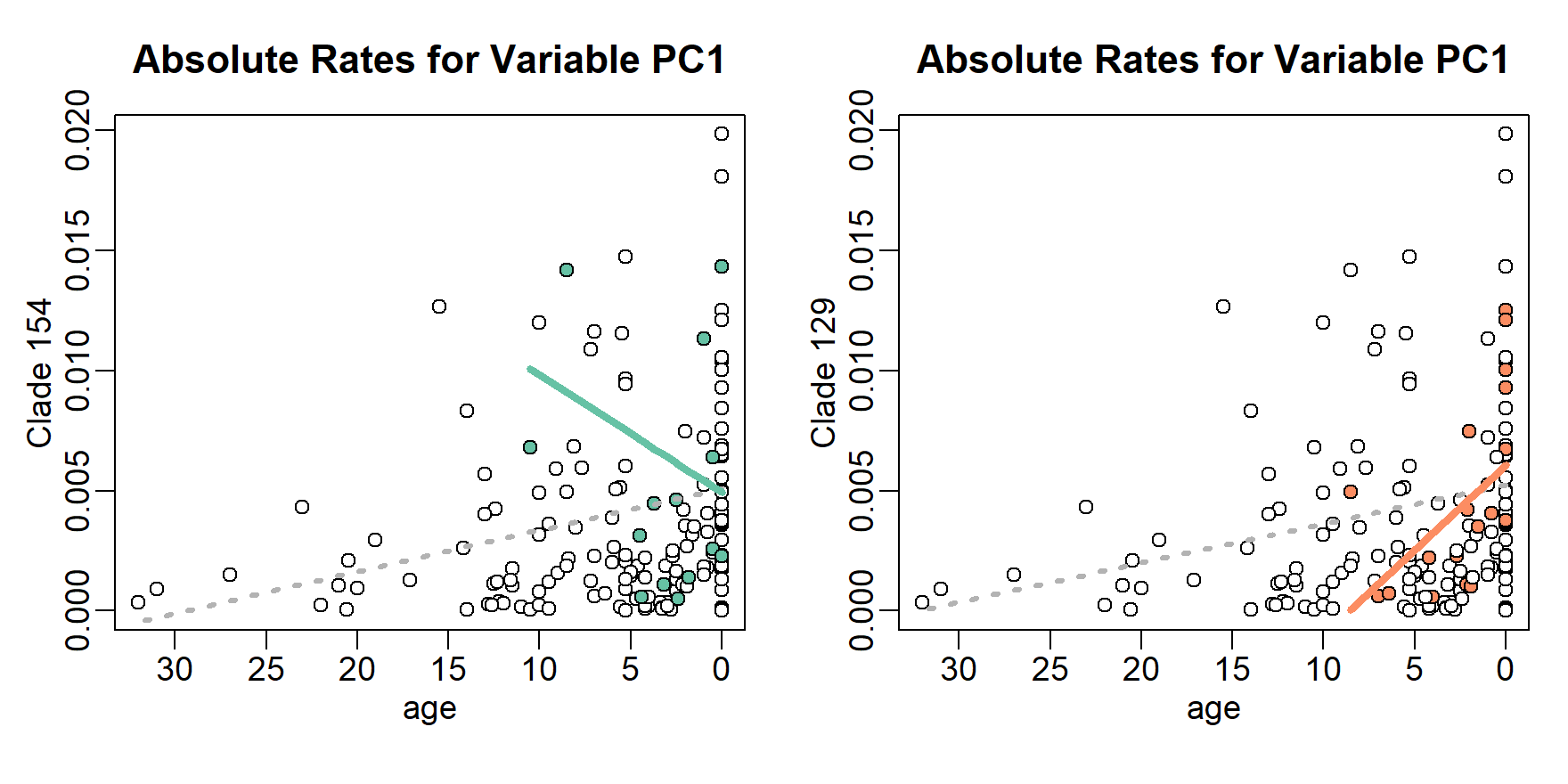``````
## Customizing parameters
par(mar=c(4,3,3,1),mgp=c(1.5,0.5,0))
pTrend2\$plotSTphenNode("PC2",node=1:2,
plot.args = list(pch.node=c(23,24),pch=1,col="gray70",cex=1.2),
lineTree.args = list(col="black",lwd=3),lineNode.args = list(lwd=5),
node.palette = c("orangered","chartreuse"))``````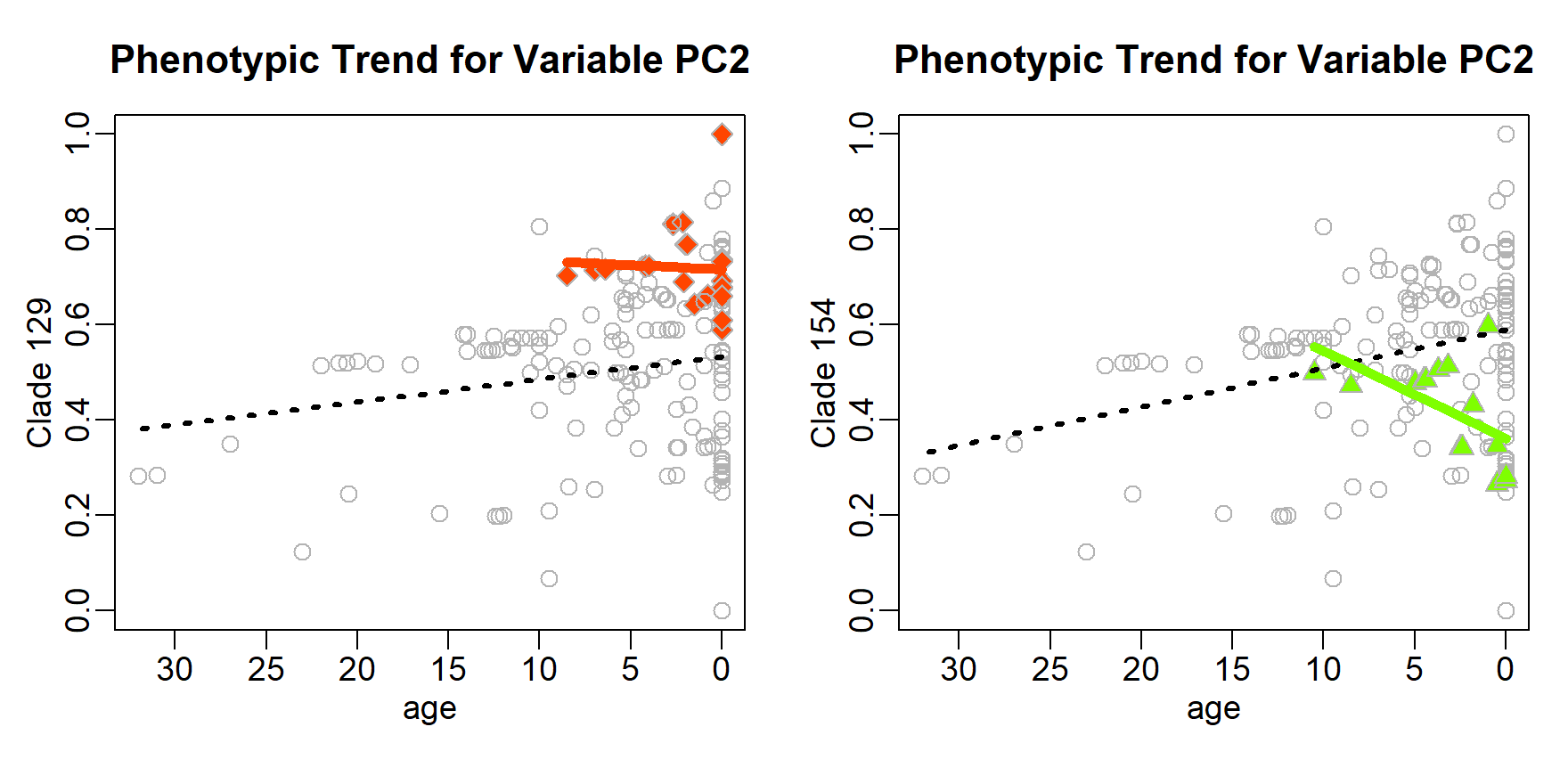``````pTrend2\$plotSTratesNode("rate",node=c(154,129),
plot.args = list(pch.node=c(5,6),pch=16,col="gray70",cex=1.2,lwd=2),
lineTree.args = list(col="gold",lwd=3,lty=4),
lineNode.args = list(lwd=5),
node.palette = c("deeppink","cyan2"))``````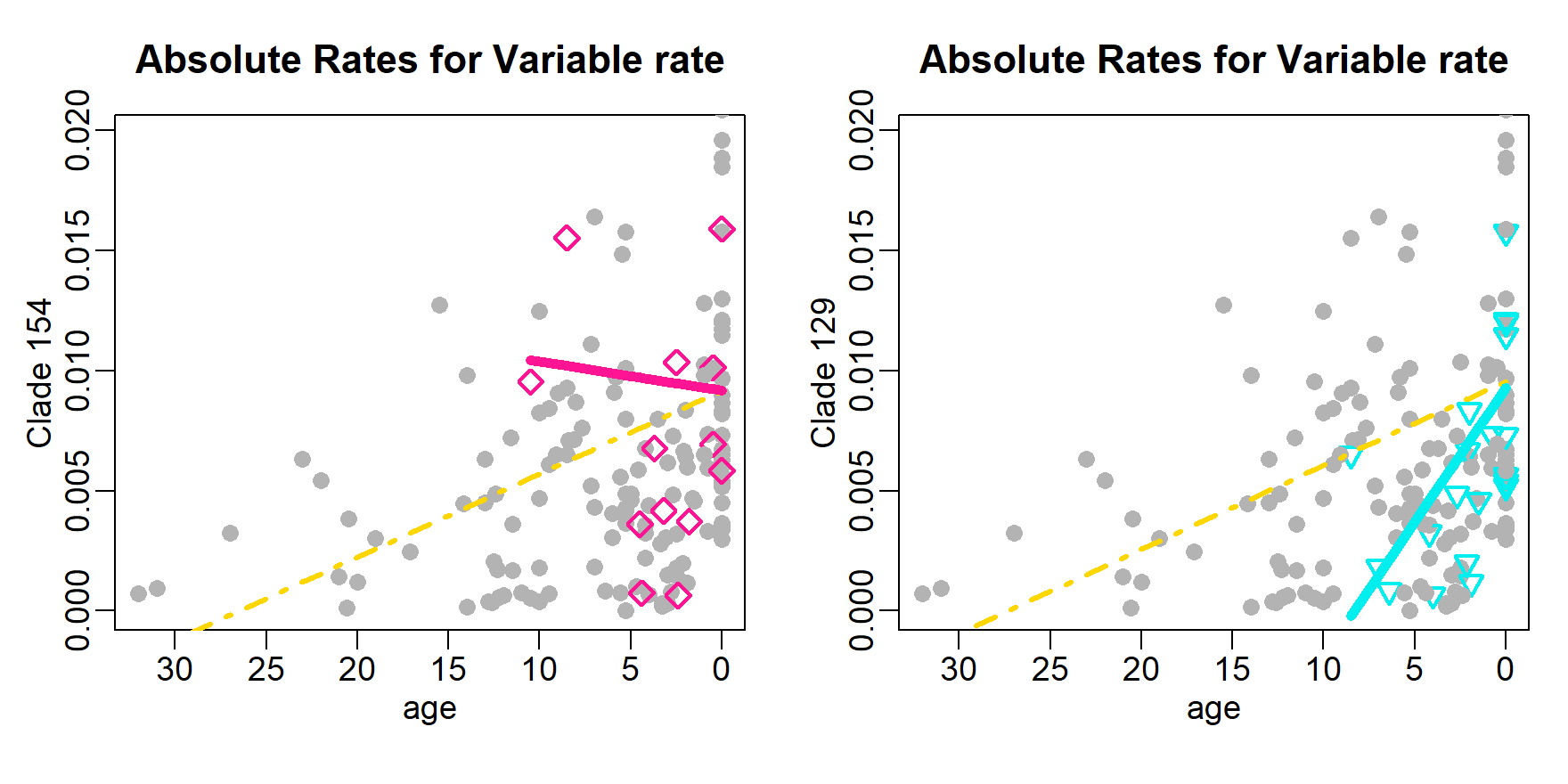## plotting search.shift results

Evolutionary rate shifts located on the tree by the function search.shift can be visualized by means of `plotShift`. The function takes as mandatory objects the outputs of `RRphylo` and `search.shift` and returns customized functions to produce plots of evolutionary rates shifts occurring at clade level (`plotClades`) or sparse across the tree (`plotStates`), depending on the `status.type` set when performing `search.shift`. In the latter case (i.e. `status.type = "sparse"`) a further `state` argument must be provided to `plotShift`.

`plotClades` highlights the shifting clades onto the phylogenetic tree by drawing colored circles on their Most Recent Common Ancestors (MRCA). The radii of such circles are directly proportional to the absolute value of absolute rate difference (see search.shift for further details), so that a circle for a clade whose mean absolute rates “shifts” more from the mean absolute rates of the tree is larger than a circle for a clade with a smaller difference. Plots for the tree and the circles can be customized by the user by specifying the arguments `tree.args` and `symbols.args`, respectively. For example, the user can choose different color options than the standard blue/red for positive/negative shifts, by setting `symbols.args=list(fg=c(pos="color for positive shift",neg="color for negative shift"))`, or provide a vector of as many colors as the number of shifting clades. The same applies to the `symbols` argument “bg”.

``````### load the RRphylo example dataset including Ornithodirans tree, body mass and locomotory type
data("DataOrnithodirans")
DataOrnithodirans\$treedino->treedino
DataOrnithodirans\$massdino->massdino
DataOrnithodirans\$statedino->statedino

# Perform RRphylo and search.shift under status.type="clade"
RRphylo(tree=treedino,y=massdino)->dinoRates

# Visualize search.shift results
plotShift(RR=dinoRates,SS=SSauto)->plotSS``````
``````# Using default options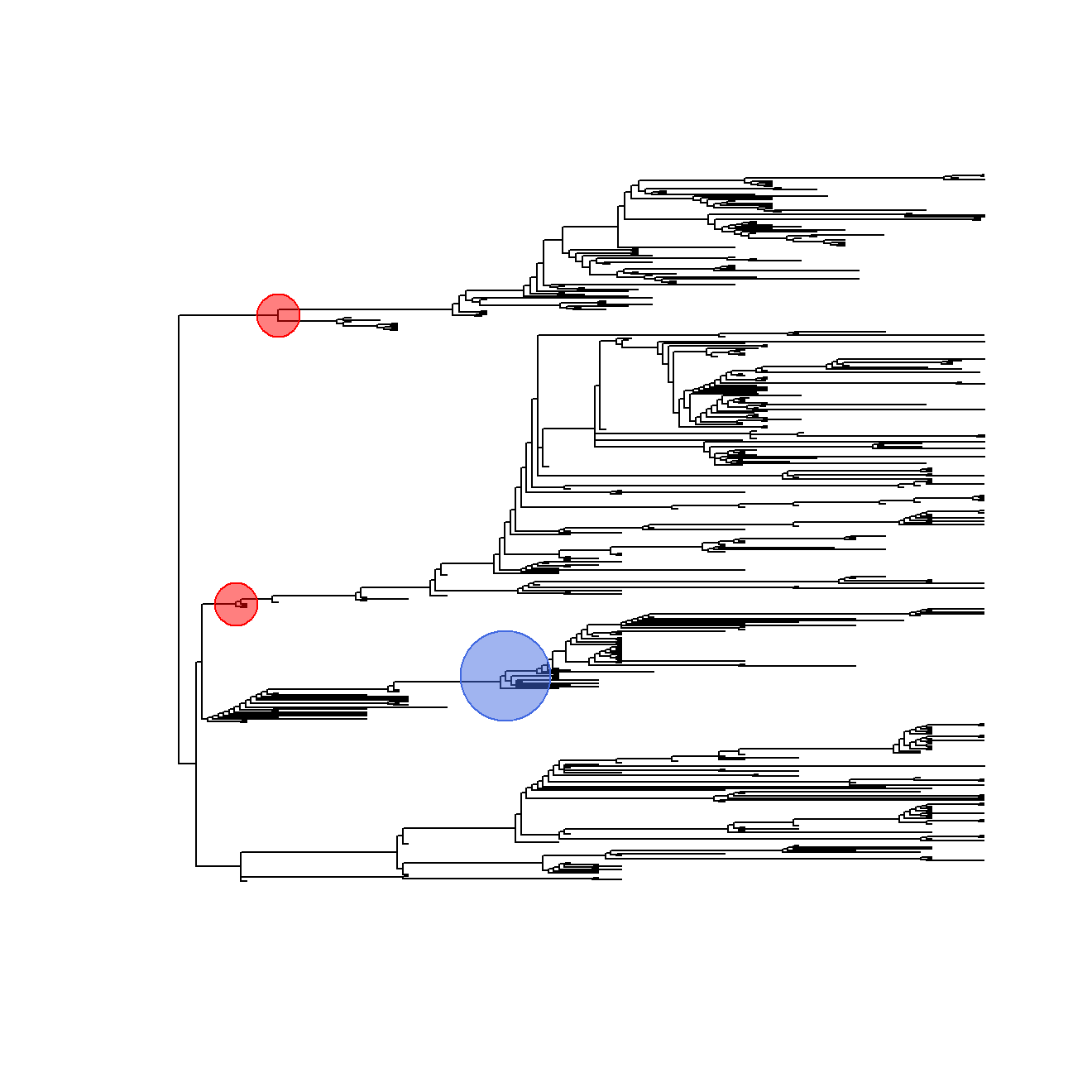``````
## Customizing parameters
# Setting different colors for positive and negative shift
symbols.args=list(lwd=2,fg=c(pos="gold",neg="green"),
bg=scales::alpha("grey70",0.3)))``````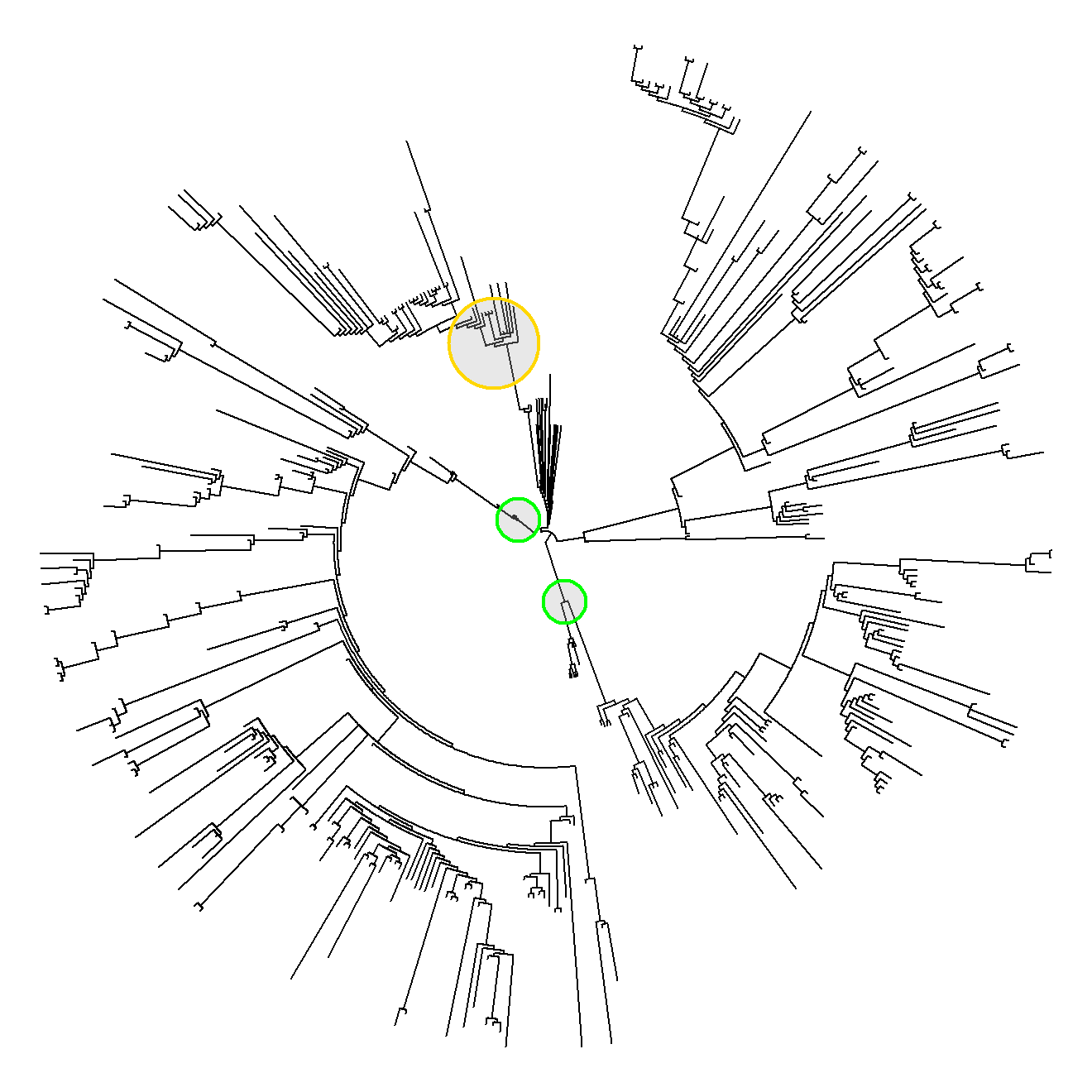``````
# Setting different colors for each shifting clade
symbols.args=list(lwd=2,fg=NA,bg=scales::alpha(c("red","green","blue"),0.3)))``````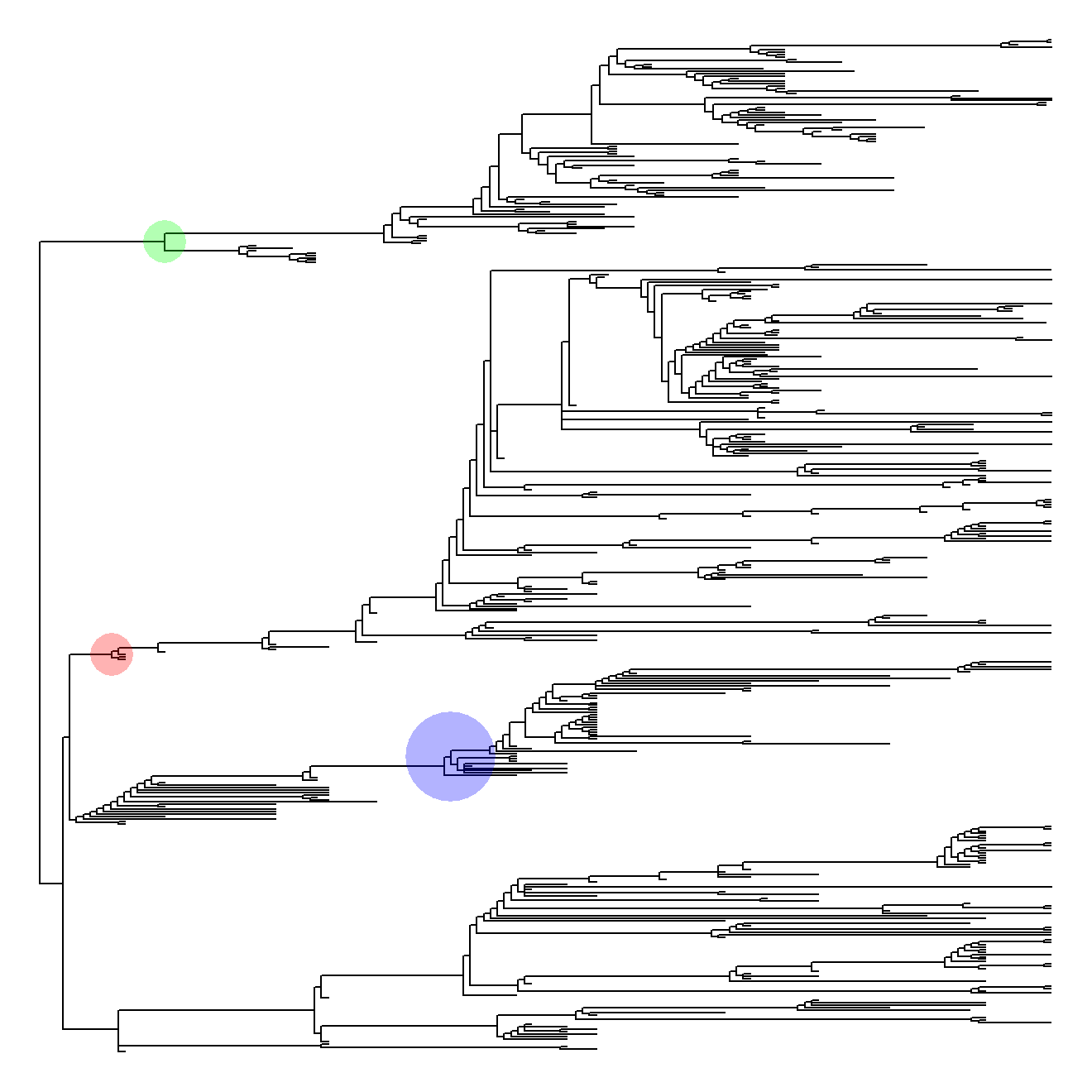If `search.shift` was performed under `status.type = "sparse"`, the function `plotStates` (returned by `plotShift`) plots the states onto the phylogenetic tree and prints into the legend the direction (i.e. positive or negative) of significant shifts for each state. Plots for the tree, the points, and the legend can be customized by the user by specifying the arguments `tree.args`, `points.args`, and `legend.args` respectively.

``````# Perform RRphylo and search.shift under status.type="sparse"
search.shift(RR=dinoRates,status.type= "sparse",state=statedino)->SSstate

# Visualize search.shift results
plotShift(RR=dinoRates,SS=SSstate,state=statedino)->plotSS2``````
``````# Using default options
plotSS2\$plotStates()``````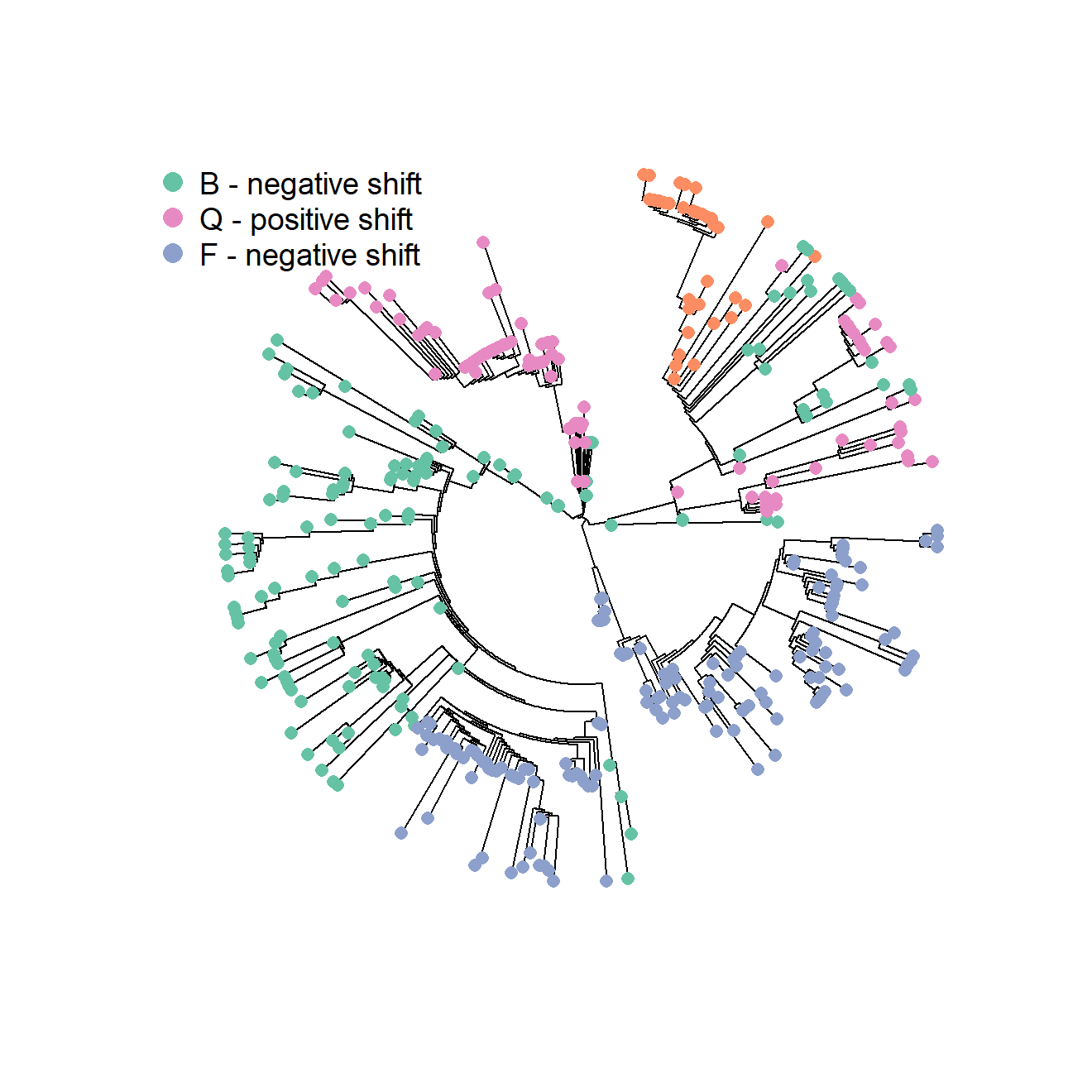``````
## Customizing parameters
# Setting customized colors and pch for different states and suppressing the legend
plotSS2\$plotStates(tree.args=list(no.margin=TRUE,type="phylogram"),
points.args=list(bg=c("gold","forestgreen","royalblue","white"),
col=c("black","black","black","orangered"),
pch=c(21,22,24,11)),legend.args=NULL)

# Setting customized colors and pch for different states and suppressing the legend
plotSS2\$plotStates(tree.args=list(no.margin=TRUE,type="phylogram"),
points.args=list(bg=c("gold","forestgreen","royalblue","white"),
col=c("black","black","black","orangered"),
pch=c(21,22,24,11)),legend.args=list(pch=21))``````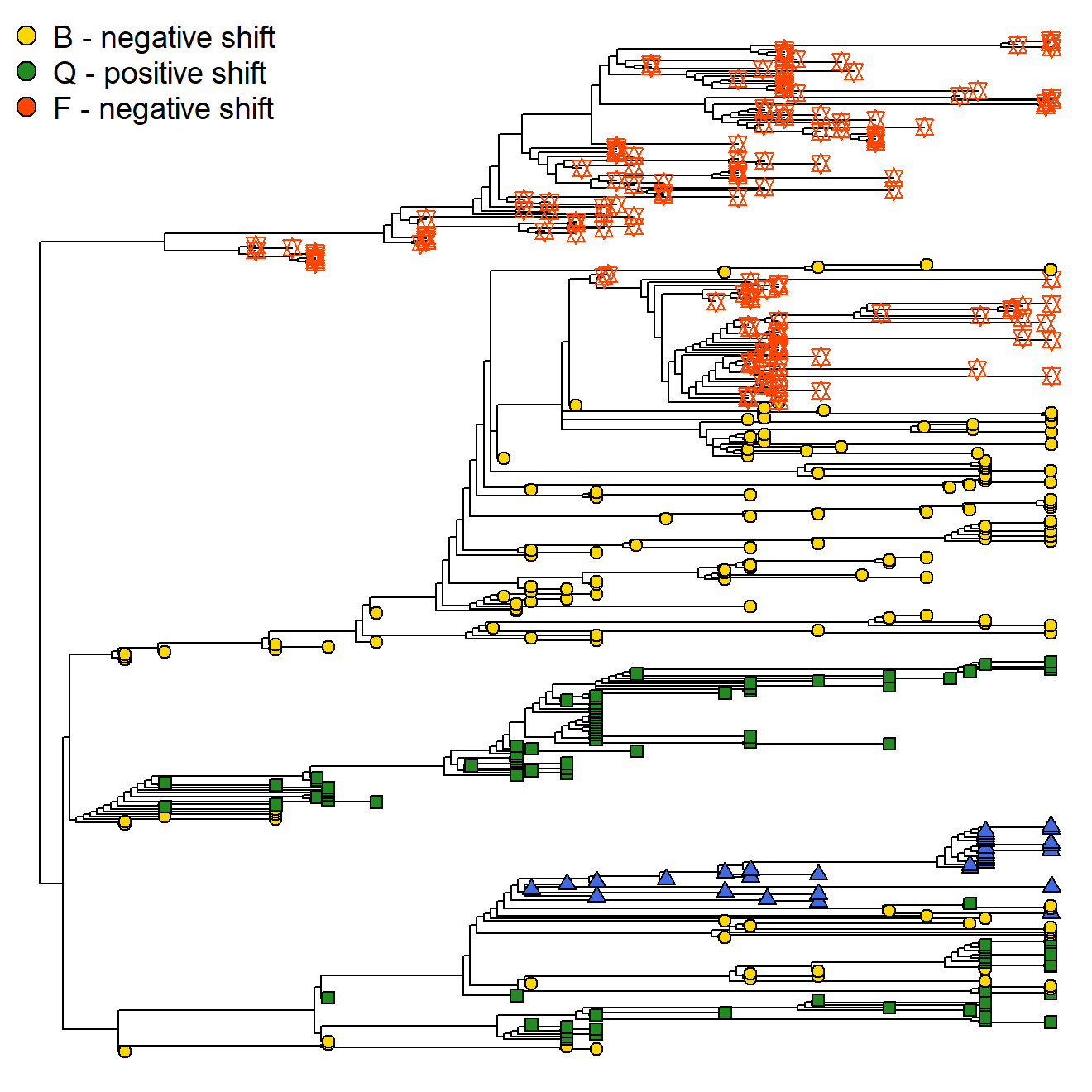## plotting search.conv results

Instances of phenotypic convergence detected on the tree by the function search.conv can be visualized by means of `plotConv`. The function takes as mandatory objects the outputs of `search.conv`, the multivariate phenotype (`y`) used to perform `search.conv`, and the index of result to plot (`variable`), and returns customized functions to produce plots of convergence occurring at clade level or related to some discrete category scattered within the tree, depending on the way `search.conv` was performed. In the latter case (convergence within/between states) a further `state` argument must be provided to `plotConv`.

When convergence between clades is inspected, four functions are returned. `plotHistTips` and `plotHistAces` generate histograms of the euclidean distances between phenotypic vectors of all possible pairs of tips/nodes within the phylogeny, and draw a vertical line representing the real distance between tips/nodes within convergent clade pair (it is the mean distance in the case of tips). Histogram and line characteristics can be customized by the user by specifying the arguments `hist.args` and `line.args`. The function `plotPChull` generates a PC1/PC2 plot (obtained by performing a PCA of the species phenotypes) with convergent clades represented by colored convex hulls, and the mean phenotype and the ancestral characters for such clades indicated by customizable symbols. The arguments passed to `plotPChull` allow to modify scatterplot elements (`plot.args`), convex hull charateristics (`chull.args`), and the points for mean phenotypes (`means.args`) and ancestral characters (`ace.args`). The function `plotTraitgram` produces a modified traitgram plot (see package picante) where converging clades are highlighted by different colors. The colors for branches belonging to converging clades and for other branches can be customized by indicating the arguments `colNodes` and `colTree`, respectively.

``````### load the RRphylo example dataset including Felids tree, 4 mandible shape variables (PCs),
### and a category indicating whether or not the species shows sabertooth morphology
data("DataFelids")
DataFelids\$PCscoresfel->PCscoresfel
DataFelids\$treefel->treefel
DataFelids\$statefel->statefel

# Perform RRphylo and search.conv between a pair of clades
cc<-2/parallel::detectCores()
RRphylo(treefel,PCscoresfel,clus=cc)->RRfel
``````# Using default options